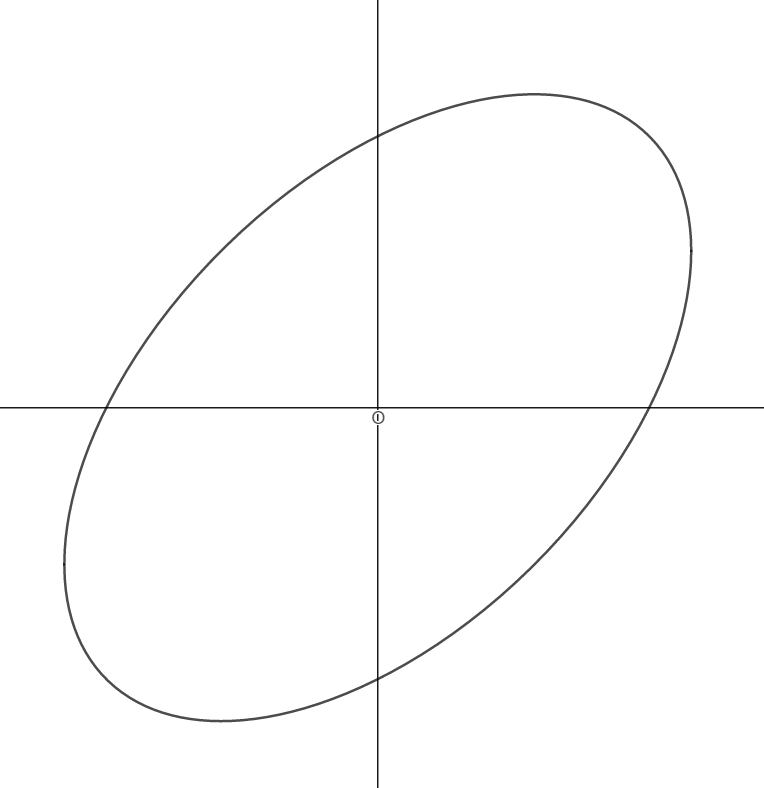# Coordinated Polygons IIIThe figure above shows a conic section with the equation $x^2+y^2-xy-9=0$. $O$ is the origin. Find the area of the quadrilateral formed when all the points intersecting the horizontal and vertical axes are joined together with straight lines.

×